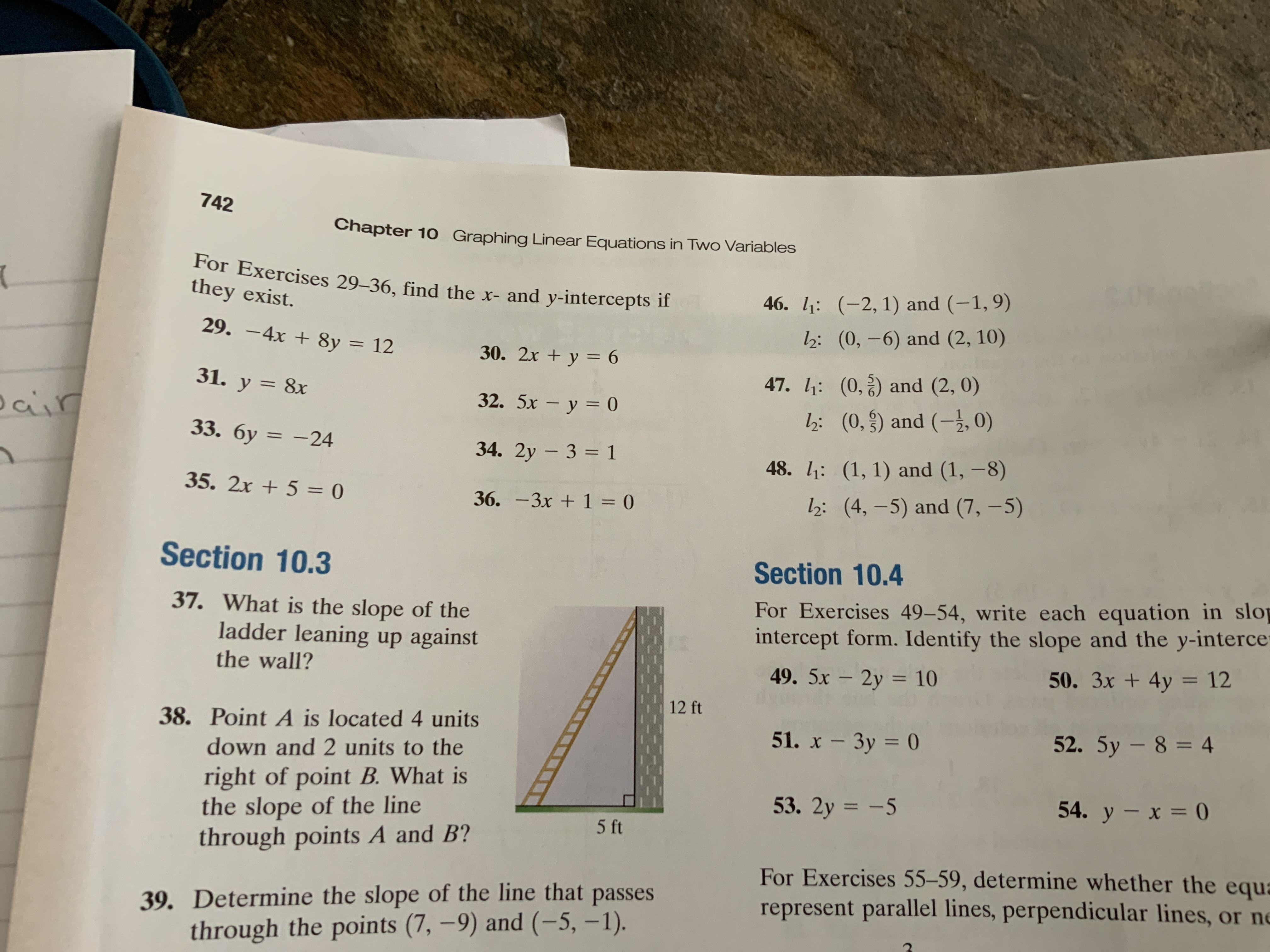# 742 Chapter 10 Graphing Linear Equations in Two Variables For Exercises 29-36, find the x- and y-intercepts if they exist. 46. : (-2, 1) and (-1,9) h: (0,-6) and (2, 10) 29. -4x +8y = 12 30. 2x y 6 47. : (0,) and (2, 0) 31. y 8x 32. 5x - y 0 ais h: (0,) and (-2, 0) 33. бу — -24 34. 2y 3 1 48. : (1, 1) and (1,-8) 35. 2x+5 = 0 h: (4, -5) and (7,-5) 36. -3x 1 = 0 Section 10.3 Section 10.4 For Exercises 49-54, write each equation in slop intercept form. Identify the slope and the y-interce 49. 5x 2y 10 37. What is the slope of the ladder leaning up against 50. 3x + 4y 12 the wall? 12 ft 38. Point A is located 4 units 52. 5y- 8=4 51. x -3y 0 down and 2 units to the right of point B.What is the slope of the line through points A and B? 53. 2y -5 54. у - х 3D0 5 ft For Exercises 55-59, determine whether the equa represent parallel lines, perpendicular lines, or ne 39. Determine the slope of the line that passes through the points (7,-9) and (-5, -1).

Question

I am Un sure how to answer number 30help_outlineImage Transcriptionclose742 Chapter 10 Graphing Linear Equations in Two Variables For Exercises 29-36, find the x- and y-intercepts if they exist. 46. : (-2, 1) and (-1,9) h: (0,-6) and (2, 10) 29. -4x +8y = 12 30. 2x y 6 47. : (0,) and (2, 0) 31. y 8x 32. 5x - y 0 ais h: (0,) and (-2, 0) 33. бу — -24 34. 2y 3 1 48. : (1, 1) and (1,-8) 35. 2x+5 = 0 h: (4, -5) and (7,-5) 36. -3x 1 = 0 Section 10.3 Section 10.4 For Exercises 49-54, write each equation in slop intercept form. Identify the slope and the y-interce 49. 5x 2y 10 37. What is the slope of the ladder leaning up against 50. 3x + 4y 12 the wall? 12 ft 38. Point A is located 4 units 52. 5y- 8=4 51. x -3y 0 down and 2 units to the right of point B.What is the slope of the line through points A and B? 53. 2y -5 54. у - х 3D0 5 ft For Exercises 55-59, determine whether the equa represent parallel lines, perpendicular lines, or ne 39. Determine the slope of the line that passes through the points (7,-9) and (-5, -1). fullscreen

1 Rating

### Want to see this answer and more?

Experts are waiting 24/7 to provide step-by-step solutions in as fast as 30 minutes!*

*Response times may vary by subject and question complexity. Median response time is 34 minutes for paid subscribers and may be longer for promotional offers.
Tagged in
Math
Algebra

### Equations and In-equations